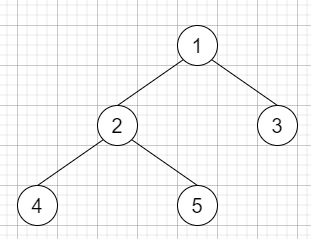# Find Leaves of Binary Tree in C++

C++Server Side ProgrammingProgramming

Suppose we have a binary tree. We will collect and remove all leaves and repeat until the tree is empty.

So, if the input is likethen the output will be [[4,5,3],,]

To solve this, we will follow these steps −

• Define one map sz

• Define one 2D array ret

• Define a function dfs(), this will take node,

• if node is null, then −

• sz[val of node] := 1 + maximum of dfs(left of node) and dfs(right of node)

• if size of ret < sz[val of node], then −

• Define an array temp

• insert temp at the end of ret

• insert val of node at the end of ret[sz[val of node] - 1]

• return sz[val of node]

• From the main method, do the following −

• dfs(root)

• return ret

## Example

Let us see the following implementation to get better understanding −

Live Demo

#include <bits/stdc++.h>
using namespace std;
void print_vector(vector<vector<auto< > v){
cout << "[";
for(int i = 0; i<v.size(); i++){
cout << "[";
for(int j = 0; j <v[i].size(); j++){
cout << v[i][j] << ", ";
}
cout << "],";
}
cout << "]"<<endl;
}
class TreeNode{
public:
int val;
TreeNode *left, *right;
TreeNode(int data){
val = data;
left = NULL;
right = NULL;
}
};
void insert(TreeNode **root, int val){
queue<TreeNode*> q;
q.push(*root);
while(q.size()){
TreeNode *temp = q.front();
q.pop();
if(!temp->left){
if(val != NULL)
temp->left = new TreeNode(val);
else
temp->left = new TreeNode(0);
return;
}else{
q.push(temp->left);
}
if(!temp->right){
if(val != NULL)
temp->right = new TreeNode(val);
else
temp->right = new TreeNode(0);
return;
}else{
q.push(temp->right);
}
}
}
TreeNode *make_tree(vector<int< v){
TreeNode *root = new TreeNode(v);
for(int i = 1; i<v.size(); i++){
insert(&root, v[i]);
}
return root;
}
class Solution {
public:
unordered_map <int, int> sz;
vector < vector <int< > ret;
int dfs(TreeNode* node){
if(!node) return 0;
sz[node->val] = 1 + max(dfs(node->left), dfs(node->right));
if(ret.size() < sz[node->val]){
vector <int< temp;
ret.push_back(temp);
}
ret[sz[node->val] - 1].push_back(node->val);
return sz[node->val];
}
vector<vector<int<> findLeaves(TreeNode* root) {
dfs(root);
return ret;
}
};
main(){
Solution ob;
vector<int< v = {1,2,3,4,5};
TreeNode *root = make_tree(v);
print_vector(ob.findLeaves(root));
}

## Input

{1,2,3,4,5}

## Output

[[3, 5, 4, ],[2, ],[1, ],]